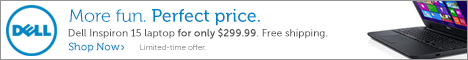## Pages## Thursday, May 22, 2008

### The Rule of 72

How long does it take for an investment to double? This is a question that is often asked, and there is a very simple formula that can be used to estimate the time it takes for your money to double. This formula is called "The Rule of 72". And the rule is:

Years to double = 72 / Interest Rate

Suppose that you were to deposit your money in the bank at an interest rate (APY) of 4%. Conceptually, you are giving the bank a loan on your money, and expect to be paid back your principle plus interest. In this example, you can expect your investment to double in 72/4 = 18 years. If you can find an interest rate of 6%, you would expect it to double in 72/6 = 12 years. The rule of 72 is also known as "the rule of 70". Using this rule, if you had an interest rate of 5%, the investment would double in 70/5 = 14 years.

At this point, I would typically go into a mathematical derivation (using such numerical concepts as natural logarithms) showing how this rule came into being. However, I will spare you the gory details this time.

One other thing is that I will mention is a similar "Rule of 115". This rule is used to estimate the time that it takes for an investment to triple in value. Similarly, the rule is:

Years to triple = 115 / Interest Rate

Again using the 5% interest rate example, it takes 14 years for your money to double. However, it would take 115/5 = 23 years to triple in value. It's all just simple math. Any questions?